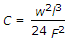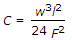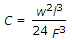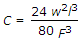# Civil Engineering - Surveying

Exercise : Surveying - Section 7
21.
Staff readings on pegs x and y from X station are 1.755 m and 2.850 m, and from station Y on staff head at Y and X are 0.655 m and 1.560 m. If reduced level of X is 105.5 m, the reduced level of Y is
104.0 m
104.5 m
105.0 m
105.5 m
Explanation:
No answer description is available. Let's discuss.

22.
The constant vertical distance between two adjacent contours, is called
horizontal interval
horizontal equivalent
vertical equivalent
contour interval
Explanation:
No answer description is available. Let's discuss.

23.
The reduced level of a floor is 99.995 m, the staff reading on the floor is 1.505 m. If the inverted staff reading against the roof is 1.795 m, the floor level below the slab, is
3.290 m
3.300 m
3.275 m
2.790 m
Explanation:
No answer description is available. Let's discuss.

24.
The correction to be applied to each 30 metre chain length along θ° slope, is
30 (sec θ - 1) m
30 (sin θ - 1) m
30 (cos θ - 1) m
30 (tan θ - 1) m
30 (cot θ - 1) m..